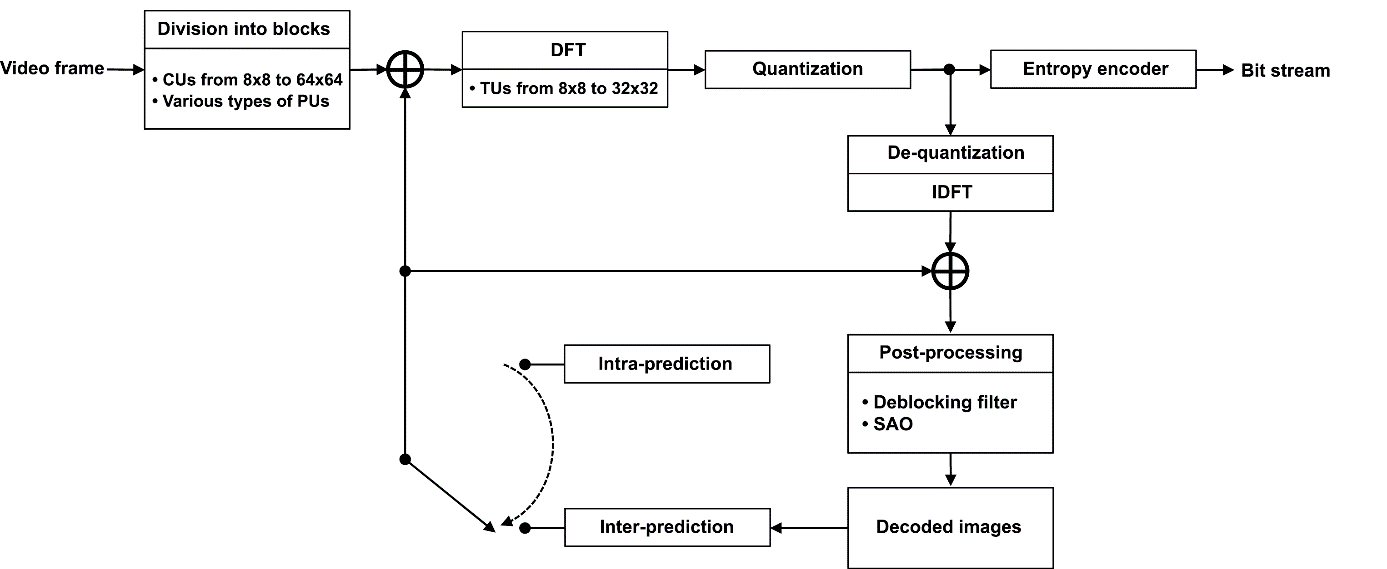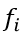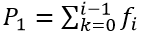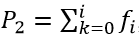### CHAPTER 6### Arithmetic coder basics and intro to binary arithmetic coder

Let us recapitulate the main steps of processing a video frame as it is being encoded using a H.265/HEVC system (Fig. 1). At the first step, termed conventionally “block division”, the frame is divided into blocks called CUs (Coding Units). The second step involves predicting the image inside each block using spatial (Intra) or temporal (Inter) prediction. When temporal prediction is being performed, a CU block can be divided into sub-blocks called PUs (Prediction Units), each of which can have its own motion vector. The predicted sample values are then subtracted from the sample values of the image being coded. A two-dimensional (2D) difference signal, or Residual signal, is formed for each CU as a result. At the third step, the 2D array of Residual signal samples is divided into so-called TUs (Transform Units), each of which undergoes a discrete 2D cosine Fourier transform (with the exception of the 4×4-sized TUs containing intra-predicted intensity samples, for which a discrete sine Fourier transform is used).Figure 1. Main steps of video frame processing in a H.265/HEVC system

At the next step, the resulting spectral Fourier coefficients are quantized by level. The data about all operations performed at each of the four steps is sent to the input of an entropy encoder; this data can be used later to restore the encoded image. This is the final step. The incoming data undergoes additional lossless compression using Context Adaptive Binary Arithmetic Coding (CABAC) algorithms.

Let us explore what this five-word sequence actually means, starting with “arithmetic coding”. To illustrate the concept of arithmetic coding, consider a simple example: compressing a message that consists of 20 characters. We will only use three possible characters: “a”, “b”, and the “EOF” character that indicates the end of the message. The message itself will look like this: {b, a, b, b, b, b, b, b, b, b, a, b, b, b, b, b, b, b, b, EOF}. The compression procedure will consist of splitting the current interval recursively. We will take [0, 1) as the initial interval and split it into smaller intervals proportional in length to the character frequencies in the message. There are 17 occurrences of the character “b” and 2 occurrences of the character “a” out of a possible 20. “EOF” occurs only once. This split will result in three intervals: [0, 2/20), [2/20, 19/20), and [19/20, 1). The first character in the message is “b”. Now we choose the interval whose length is proportional to the frequency of the character “b”, i.e. [2/20, 19/20), as the current interval. Then we split the current interval in the same way as above and choose the interval whose length is proportional to the next character’s frequency as the next current interval. This procedure is repeated until the end of the message. Let us present our coding workflow as a table.

### Table 1

 Iteration No. Current Interval Splitting Result Character in the message a b EOF 1 [0, 1) [0, 0.1) [0.1, 0.95) [0.95, 1) b 2 [0.1, 0.95) [0.1, 0.185) [0.185, 0.855) [0.855, 0.95) a 3 [0.1, 0.185) [0.1,0.1085) [0.1085,0.1808) [0.1808,0.185) b 4 [0.1085, 0.1808) [0.1085,0.1157) [0.1157,0.1771) [0.1771,0.1808) b 5 [0.1157,0.1771) [0.1157,0.1219) [0.1219,0.1741) [0.1741,0.1771) b 6 [0.1219,0.1741) [0.1219,0.1271) [0.1271,0.1715) [0.1715,0.1741) b 7 [0.1271,0.1715) [0.1271,0.1315) [0.1315,0.1692) [0.1692,0.1715) b 8 [0.1315,0.1692) [0.1315,0.1353) [0.1353,0.1674) [0.1674,0.1692) b 9 [0.1353,0.1674) [0.1353,0.1385) [0.1385,0.1657) [0.1657,0.1674) b 10 [0.1385,0.1657) [0.1385,0.1412) [0.1412,0.1644) [0.1644,0.1657) b 11 [0.1412,0.1644) [0.1412,0.1435) [0.1435,0.1562) [0.1562,0.1644) a 12 [0.1412,0.1435) [0.1412,0.1415) [0.1415,0.1434) [0.1434,0.1435) b 13 [0.1415,0.1434) [0.1415,0.1417) [0.1417,0.1433) [0.1433,0.1434) b 14 [0.1417,0.1433) [0.1417,0.1418) [0.1418,0.1432) [0.1432,0.1433) b 15 [0.1418,0.1432) [0.1418,0.1420) [0.1420,0. 14317) [0.14317,0.1432) b 16 [0.1420,0. 14317) [0.1420,0.1421) [0.1421,0.14311) [0.14311,0.14317) b 17 [0.1421,0.14311) [0.1421,0.1422) [0.1422,0.14306) [0.14306,0.14317) b 18 [0.1422,0.14306) [0.1422,0.1423) [0.1423,0.14302) [0.14302,0.14306) b 19 [0.1423,0.14302) [0.1423,0.14235) [0. 14235,0.14298) [0.14298,0. 14302) b 20 [0. 14235,0.14298) [0.14235,0.14241) [0.14241,0.14295) [0.14295,0.14298) EOF

The recursive splitting of the current interval has resulted in the interval that is bolded in the table, which we will show here in the unrounded form: [0.142948471255693, 0.142980027967343). In the binary notation, this interval is represented as [0.001 001 001 001 100 00100010101100001000011100111000000010, 0.001 001 001 001 101 00101011011010000000110011101010011101). The number 0.001 001 001 001 100 1, corresponding to decimal 0.142959594726563 and included in the resulting interval, is the encoded message. It is a 16-bit value. Thus, a 20-character long message has been converted into a 16-bit code. We have compressed our message!

Now let us try to decode it. Again, we will take [0, 1) as the initial interval and split it according to the character frequencies in the message. Obviously, the result of this split is shown in Table 1 in the line where the Iteration No. is 1. The interval that contains the number 0.142959594726563 is the middle interval [0.1, 0.95), therefore the first decoded character is “b” (which is reflected by the 5th column in the first table line). [0.1, 0.95) now becomes the current interval, which we again split into three parts according to the character frequency in the message. The result is shown in the second line of Table 1. The number 0.142959594726563 belongs to the first of the resulting intervals, [0.1, 0.185). The length of this interval is proportional to the frequency of the character “a”, therefore “a” is the next decoded character. It is evident from the above that the entire decoding process is already described by Table 1. The iterative splitting of the current interval will continue until the character “EOF” is decoded, indicating the end of the message.

Although these iterative procedures of splitting the current interval do in fact implement arithmetic encoding and decoding of a message, they bear little similarity to how encoding/decoding is actually done when using CABAC algorithms. This is primarily due to two significant practical shortcomings of the encoding and decoding procedures described above. Firstly, we only obtained the encoding result after having processed the entire message. Not a single bit of the result was known prior to that. Similarly, to start decoding, we need to know the entire bit sequence that represents the encoded message. The second shortcoming is also quite apparent from our example. When the current interval is split iteratively, each iteration increases the precision required to represent the interval endpoints. Therefore, the longer the message, the longer the time period (delay) needed to process it in the encoder and decoder, and the higher the precision (processing bit width) required to implement arithmetic coding algorithms.

Let us point out several fairly obvious considerations related to the encoding procedure. Clearly, if the current interval is fully contained inside the area between 0 and ½, the current bit of the encoding result will be zero. In a similar fashion, if the current interval is fully contained inside the area between ½ and 1, the current bit of the encoding result will have a value of one. If, however, the left endpoint of the current interval is less than ½ and the right is greater than ½, but both differ from ½ by no more than ¼, the current result bit will be unknown. On the other hand, it can be said with certainty that the next result bit will have an opposite value to the current bit. When we have obtained each current result bit for this iteration, we can then double the interval length. All of this made it possible to introduce a procedure for doubling the current interval length that overcomes both the abovementioned shortcomings of arithmetic coding.

This procedure is called Renormalization in the standard. With Renormalization, the encoding result bits are output immediately during encoding (before it is finished), and the current interval length is doubled. Renormalization is carried out iteratively each time a new current interval is selected. The iterations proceed until the current interval is fully contained inside one of the following three intervals: [0, 0.5), [0.25, 0.75), [0.5, 1). If the current interval is not fully contained inside any of these, the iterations stop. Otherwise, if the current interval is within one of the three intervals, one of three processing sequences is executed, each of which corresponds to a specific interval.

If the entire current interval is contained inside the interval [0, 0.5), 0 is output as the result bit, followed by as many 1-valued bits as have been accumulated when processing the previous characters. (The number of ones output to the resulting bit stream is equal to the value of the counter that has the name bitsOutstanding in the standard. After the 1-valued bits have been output, the counter is reset to 0). The endpoint values of the current interval are doubled. (As a result, the length of the interval also gets doubled; for the sake of brevity, let us call this doubling “interval expansion to the right”.)

If the entire current interval is contained inside the interval [0.5, 1), 1 is output as the result bit, followed by as many 0-valued bits as have been accumulated when processing the previous characters. (Again, the number of zeros is equal to the value of the bitsOutstanding counter, which is reset to 0 after this operation.) The interval endpoints are moved to the left so as to double the distance from them to point 1. (We will call this “interval expansion to the left”.)

If the current interval is fully contained inside the interval [0.25, 0.75), this fact needs to be memorized for subsequent result bit output (increment the bitsOutstanding counter by 1). The left interval endpoint is moved to the left so as to double the distance from it to point 0.5. The right interval endpoint is moved to the right, with the distance from it to point 0.5 also being doubled. (We will call this doubling of the interval “interval expansion to both sides”.)

In addition, let us formalize the procedure of selecting the two last bits of the encoded message that governs the selection of a specific binary value from an interval obtained by iterative splitting. This procedure is very simple: if the left endpoint of the resulting interval is less than 0.25, then the last two message bits are {0, 1}, otherwise they are {1, 0}.

To illustrate how the renormalization procedure works, we will use the example of encoding the same 20-character message: {b, a, b, b, b, b, b, b, b, b, a, b, b, b, b, b, b, b, b, EOF}.

### Table 2

 Iteration No. Current Interval Splitting Result Character in the message a b EOF 1 [0, 1) [0, 0.1) [0.1, 0.95) [0.95, 1) b 2 [0.1, 0.95) [0.1, 0.185) [0.185, 0.855) [0.855, 1) a [0.1, 0.185) Output 0, expand the interval to the right [0.2, 0.37) Output 0, expand the interval to the right [0.4, 0.74) Increment the counter, expand the interval to both sides 3 [0.3, 0.98) [0.3, 0.368) [0.368, 0.946) [0.946, 0.98) b 4 [0.368, 0.946) [0.368,0.4258) [0.4258, 0.9171) [0.9171, 0.946) b 5 [0.4258, 0.9171) [0.4258, 0.4749) [0.4749, 0.8925) [0.8925, 0.9171) b 6 [0.4749, 0.8925) [0.4749, 0.5167) [0.5167, 0.8717) [0.8717, 0.8925) b [0.5167, 0.8717) output 10, expand the interval to the left, reset the counter to 0 7 [0.0334, 0.7433) [0.0334, 0.1044) [0.1044, 0.7078) [0.7078, 0.7433) b 8 [0.1044, 0.7078) [0.1044, 0.1647) [0.1647, 0.6776) [0.6776, 0.7078) b 9 [0.1647, 0.6776) [0.1647, 0.2160) [0.2160, 0.6520) [0.6520, 0.6776) b 10 [0.2160, 0.6520) [0.2160, 0.2596) [0.2596, 0.6302) [0.6302, 0.6520) b [0.2596, 0.6302) increment the counter, expand the interval to both sides 11 [0.0192, 0.7604) [0.0192, 0.0933) [0.0933, 0.7233) [0.7233, 0.7604) a [0.0192, 0.0933) output 01, expand the interval to the right, reset the counter [0.0384, 0.1867) output 0, expand the interval to the right [0.0769, 0.3733) output 0, expand the interval to the right 12 [0.1537, 0.7467) [0.1537, 0.2130) [0.2130, 0.7170) [0.7170, 0.7467) b 13 [0.2130, 0.7170) [0.2130, 0.2634) [0.2634, 0.6918) [0.6918, 0.7170) b [0.2634, 0.6918) increment the counter, expand the interval to both sides 14 [0.0269, 0.8837) [0.0269, 0.1125) [0.1125, 0.8408) [0.8408, 0.8837) b 15 [0.1125, 0.8408) [0.1125, 0.1854) [0.1854, 0.8044) [0.8044, 0.8408) b 16 [0.1854, 0.8044) [0.1854, 0.2473) [0.2473, 0.7735) [0.7735, 0.8044) b 17 [0.2473, 0.7735) [0.2473, 0.2999) [0.2999, 0.7471) [0.7471, 0.7735) b [0.2999, 0.7471) increment the counter, expand the interval to both sides 18 [0.0998, 0.9943) [0.0998, 0.1892) [0.1892, 0.9496) [0.9496, 0.9943) b 19 [0.1892, 0.9496) [0.1892, 0.2653) [0.2653, 0.9115) [0.9115, 0.9496) b 20 [0.2653, 0.9115) [0.2653, 0.3299) [0.3299, 0.8792) [0.8792, 0.9115) EOF [0.8792, 0.9115) output 100, expand the interval to the left, reset the counter to 0 [0.7585, 0.8231) output 1, expand the interval to the left [0.5169, 0.6462) output 1, expand the interval to the left [0.0339, 0.2924) output 0, expand the interval to the right [0.0678, 0.5848) since 0.0678 < 0.25, output 01

The result of encoding is 001 001 001 001 100 1. These are the same 16 bits that we obtained before without using the Renormalization procedure.

Let us note one more thing here. The procedure of iterative splitting of the current interval into parts proportional in length to the character frequencies in the message can be easily formalized. Indeed, if we denote the relative frequency of the i-th character as(in our example, this equals 0.1 for the first character, “a”), introduce the definitionsand, then the endpoints of the current interval at each iteration can be calculated as:

R = Hc - Lc

L = Lc + P1 ∙ R ,

H = Lc + P2 ∙ R ,

где Lc – left endpoint of the current interval, L – is the new value of the left endpoint of the current interval after splitting, Hc– right endpoint of the current interval, H – is the new right endpoint value of the current interval after splitting.

May 13, 2019

Chapter 1. Video encoding in simple terms

Chapter 2. Inter-frame prediction (Inter) in HEVC

Chapter 3. Spatial (Intra) prediction in HEVC

Chapter 4. Motion compensation in HEVC

Chapter 5. Post-processing in HEVC